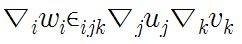## Homework Statement

Let us consider three scalar fields u(x), v(x), and w(x). Show that they have a relationship such that f(u, v, w) = 0 if and only if

(∇u) × (∇v) · (∇w) = 0.

## The Attempt at a Solution

I could think nothing but just writing the components of (∇u) × (∇v) · (∇w) = 0. How could i prove this?

Last edited:

## Homework Statement

Let us consider three scalar fields u(x), v(x), and w(x).
Show that they have a relationship such that f(u, v, w) = 0 if and only if
(∇u) × (∇v) · (∇w) = 0.

## The Attempt at a Solution

I can do nothing but just writing components of (∇u) × (∇v) · (∇w). How can I prove this identity?

Ray Vickson
Homework Helper
Dearly Missed

## Homework Statement

Let us consider three scalar fields u(x), v(x), and w(x). Show that they have a relationship such that f(u, v, w) = 0 if and only if

(∇u) × (∇v) · (∇w) = 0.

## The Attempt at a Solution

I could think nothing but just writing the components of (∇u) × (∇v) · (∇w) = 0. How could i prove this?

If ##\vec{A} = \nabla u##, ##\vec{B} = \nabla v## and ##\vec{C} = \nabla w##, what does the given condition say about the directions of the vectors ##\vec{A}, \vec{B}, \vec{C}##?

Scalar triple product means det(a,b,c) or volume of a parallelepiped. Is it a key to solve this problem?

When you wrote the components out, what did you get?

I wroteby using levi-civita symbol

Dick
Homework Helper
Scalar triple product means det(a,b,c) or volume of a parallelepiped. Is it a key to solve this problem?

One way is easy. If f(u,v,w)=0 then to show the triple product is zero you just have to show that the gradients of u, v and w are linearly dependent. Use the chain rule. The other way is harder, you need to have some sort of integrability theorem like Frobenius. I'm a little fuzzy on that. What have you got?

Last edited:
mfb
Mentor
Sorry, am I missing something? There is always such a function f(u,v,w) - just define it to be zero everywhere. Are we looking for a non-trivial (!) linear function f?

Dick